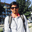Related Tags

beginners
coding challenge
leetcode
communitycreator

# How to check if the kth bit is set/unsetGopi Gorantala### Overview

We try to find a bit in the binary representation of a number at the $k^{th}$ position and check if it is set/unset.

### Problem statement

Given two positive integers (n and k) we check whether the bit at position k, from the right in the binary representation of n, is set 1 or unset 0.

Example 01:

Input: n = 5, k = 1

Output: true


Example 02:

Input: n = 10, k = 2

Output: true


Example 03:

Input: n = 10, k = 1

Output: false


### Code

if(n == 0) return;
k = 1
while(true) {
if((n & (1 << (k - 1))) == 0)
increment k
else {
return k
}
}


### Complexity analysis

Time Complexity: O(1) This always remains constant, as we are dealing with the bit representation of the decimals or ASCII. They are represented by either 32 or 64 bit.

Space Complexity: O(1) because we are not using any extra space in our code.

### Optimal solution (refactored)

class CheckKthBitSetOrNot {
private static boolean checkKthBitSet(int n, int k) {
return (n & (1 << (k - 1))) != 0;
}

public static void main(String[] args) {
System.out.println("n = 5, k = 3 : " + checkKthBitSet(5, 3));
System.out.println("------------");
System.out.println("n = 10, k = 2 : " + checkKthBitSet(10, 2));
System.out.println("------------");
System.out.println("n = 10, k = 1 : " + checkKthBitSet(10, 1));
}
}


### Explanation

We used the left shift operation to shift the bits to $k^{th}$ position and then use the & operation with number 1, and check if it is not-equals to 0.

Let’s see these in 32-bit binary representation

### Case 01

Input n = 5, k = 3

n    = 5 = 00000000 00000000 00000000 00000101
1    = 1 = 00000000 00000000 00000000 00000001
k    = 3 = 00000000 00000000 00000000 00000011
(k - 1) = 2 = 00000000 00000000 00000000 00000010

Now we'll shift 1 with (k - 1) positions. We are shifting number 1 two-bit positions to the left side.

(1 << (k - 1)) = 4 = 00000000 00000000 00000000 00000100

Now, doing the & operation of n and (1 << (k - 1)) will give us a decimal number. If that is not equal to 0, then the bit is set, and we return true.

         n         = 5 = 00000000 00000000 00000000 00000101
(1 << (k - 1))   = 4 = 00000000 00000000 00000000 00000100
-------------------------------------------------------------
n & (1 << (k - 1)) = 4 = 00000000 00000000 00000000 00000100
-------------------------------------------------------------

So, n & (1 << (k - 1)) = 4, which is not 0, so we return true.

### Complexity analysis

Time Complexity: O(1) This is constant, as we are dealing with the bit representation of the decimals or ASCII. They are represented in either 32 bit or 64 bit.

Space Complexity: O(1) because we are not using any extra space in our code.

We can solve this problem using the right shift as well. We will see how to solve the same problem using the >> operator in the next chapter.

Note: On how to solve problems using bit manipulation, you can visit it here: Master Bit Manipulation for Coding Interviews.

RELATED TAGS

beginners
coding challenge
leetcode
communitycreator

CONTRIBUTORGopi Gorantala
RELATED COURSES

View all Courses

Keep Exploring

Learn in-demand tech skills in half the time